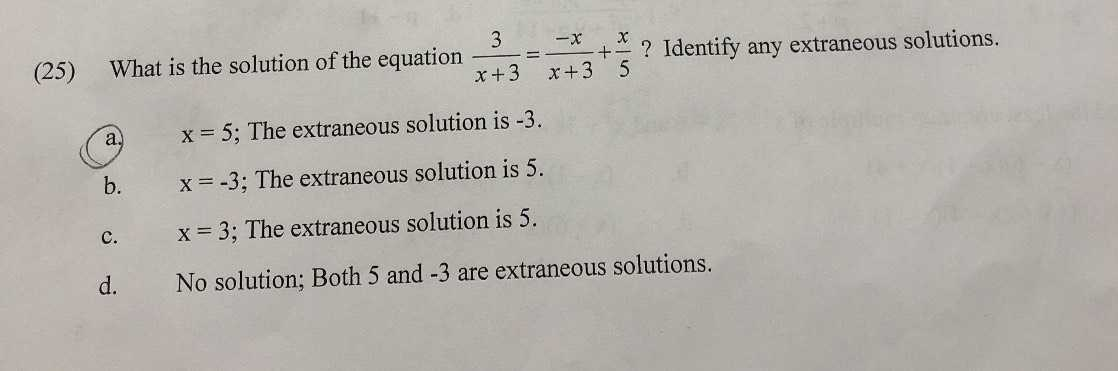### ¿Todavía tienes preguntas de matemáticas?

Pregunte a nuestros tutores expertos
Algebra
Pregunta(25) What is the solution of the equation $$\frac { 3 } { x + 3 } = \frac { - x } { x + 3 } + \frac { x } { 5 }$$ ? Identify any extraneous solutions. a. $$x = 5$$ ; The extraneous solution is $$- 3$$ . b. $$x = - 3$$ ; The extraneous solution is $$5$$ . c. $$x = 3$$ ; The extraneous solution is $$5$$ . d. Nolution; Both $$5$$ and $$- 3$$ are extraneous solutions.